Next: Fluid Equations Up: Plasma Fluid Theory Previous: Moments of the Collision

# Moments of the Kinetic Equation

We obtain fluid equations by taking appropriate moments of the ensemble-average kinetic equation, (175). In the following, we suppress all ensemble-average over-bars for ease of notation. It is convenient to rearrange the acceleration term,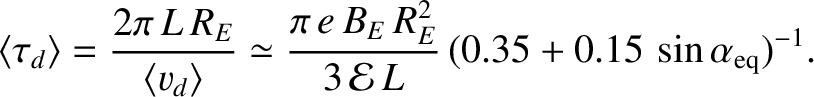(206)

The two forms are equivalent because flow in velocity space under the Lorentz force is incompressible: i.e.,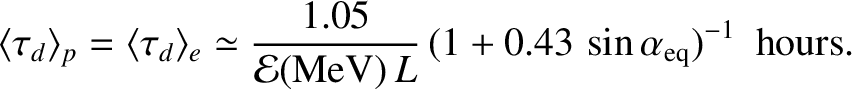(207)

Thus, Eq. (175) becomes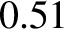(208)

The rearrangement of the flow term is, of course, trivial, since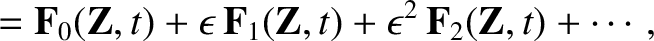is independent of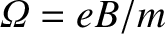.

The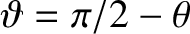th moment of the ensemble-average kinetic equation is obtained by multiplying the above equation bypowers ofand integrating over velocity space. The flow term is simplified by pulling the divergence outside the velocity integral. The acceleration term is treated by partial integration. Note that these two terms couple theth moment to the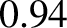th and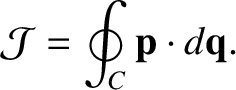th moments, respectively.

Making use of the collisional conservation laws, the zeroth moment of Eq. (208) yields the continuity equation for species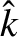: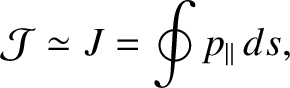(209)

Likewise, the first moment gives the momentum conservation equation for species: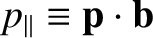(210)

Finally, the contracted second moment yields the energy conservation equation for species: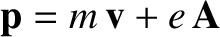(211)

The interpretation of Eqs. (209)-(211) as conservation laws is straightforward. Suppose that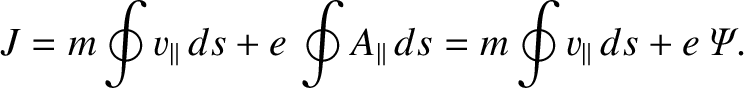is some physical quantity (e.g., total number of particles, total energy, ...), and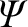is its density: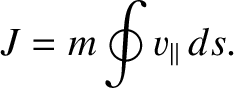(212)

Ifis conserved then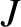must evolve according to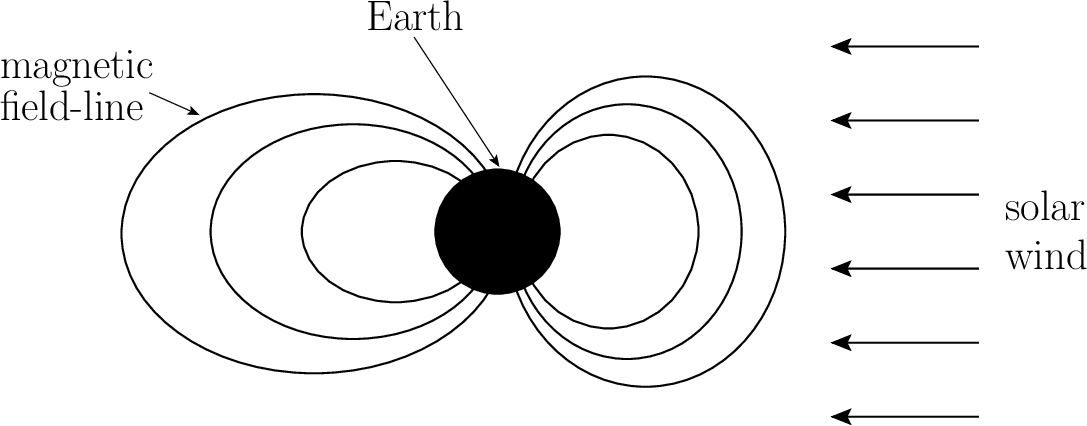(213)

where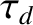is the flux density of, and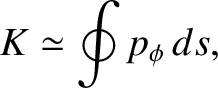is the local rate per unit volume at whichis created or exchanged with other entities in the fluid. Thus, the density ofat some point changes because there is net flow oftowards or away from that point (measured by the divergence term), or because of local sources or sinks of(measured by the right-hand side).

Applying this reasoning to Eq. (209), we see thatis indeed the species-particle flux density, and that there are no local sources or sinks of species-particles.From Eq. (210), we see that the stress tensor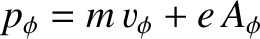is the species-momentum flux density, and that the species-momentum is changed locally by the Lorentz force and by collisional friction with other species. Finally, from Eq. (211), we see that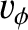is indeed the species-energy flux density, and that the species-energy is changed locally by electrical work, energy exchange with other species, and frictional heating.Next: Fluid Equations Up: Plasma Fluid Theory Previous: Moments of the Collision
Richard Fitzpatrick 2011-03-31